## DC Ammeter:

DC Ammeter – The PMMC galvanometer constitutes the basic movement of a dc ammeter. Since the coil winding of a basic movement is small and light, it can carry only very small currents. When large currents are to be measured, it is necessary to bypass a major part of the current through a resistance called a shunt, as shown in Fig. 3.1. The resistance of shunt can be calculated using conventional circuit analysis.

Referring to Fig. 3.1

• Rm = internal resistance of the movement.
• Ish = shunt current
• Im = full scale deflection current of the movement
• I = full scale current of the ammeter + shunt (i.e. total current)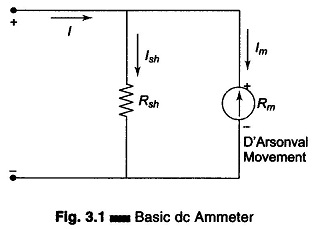Since the shunt resistance is in parallel with the meter movement, the voltage drop across the shunt and movement must be the same.

Therefore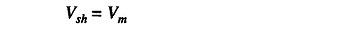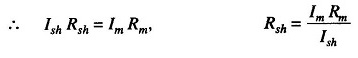But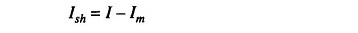hence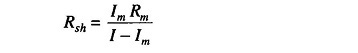For each required value of full scale meter current, we can determine the value of shunt resistance.

### Multirange Ammeters:

The current range of the dc ammeter may be further extended by a number of shunts, selected by a range switch. Such a meter is called a multirange ammeter, shown in Fig. 3.2.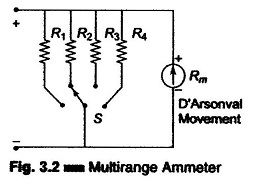The circuit has four shunts R1, R2, Rand R4, which can be placed in parallel with the movement to give four different current ranges. Switch S is a multiposition switch, (having low contact resistance and high current carrying capacity, since its contacts are in series with low resistance shunts). Make before break type switch is used for range changing. This switch protects the meter movement from being damaged without a shunt during range changing.

If we use an ordinary switch for range changing, the meter does not have any shunt in parallel while the range is being changed, and hence full current passes through the meter movement, damaging the movement. Hence a make before break type switch is used. The switch is so designed that when the switch position is changed, it makes contact with the next terminal (range) before breaking contact with the previous terminal. Therefore the meter movement is never left unprotected. Multirange ammeters are used for ranges up to 50A. When using a multirange ammeter, first use the highest current range, then de­crease the range until good upscale reading is obtained. The resistance used for the various ranges are of very high precision values, hence the cost of the meter increases.

### The Aryton Shunt or Universal Shunt:

The Aryton shunt eliminates the pos­sibility of having the meter in the cir­cuit without a shunt. This advantage is gained at the price of slightly higher overall resistance. Figure 3.3 shows a circuit of an Aryton shunt ammeter. In this circuit, when the switch is in position “1”, resistance Ra is in parallel with the series combination of Rb, Rc, and the meter movement. Hence the current through the shunt is more than the current through the meter movement, thereby protecting the meter movement and reducing its sensitivity. If the switch is connected to position “2”, resistance Ra and Rb are together in parallel with the series combination of Rc and the meter movement. Now the current through the meter is more than the current through the shunt resistance.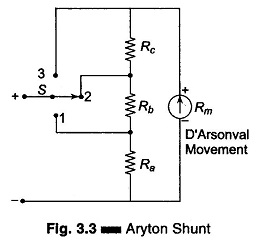If the switch is connected to position “3” Ra, Rb and Rc are together in parallel with the meter. Hence, maximum current flows through the meter movement and very little through the shunt. This increases the sensitivity.

### Requirements of a Shunt:

The type of material that should be used to join the shunts should have two main properties.

1. Minimum Thermo Dielectric Voltage Drop

Soldering of joint should not cause a voltage drop.

2. Solderability

Resistance of different sizes and values must be soldered with minimum change in value.

The following precautions should be observed when using an ammeter for measurement.

1. Never connect an ammeter across a source of emf. Because of its low resistance it would draw a high current and destroy the movement. Always connect an ammeter in series with a load capable of limiting the current.

2. Observe the correct polarity. Reverse polarity causes the meter to deflect against the mechanical stopper, which may damage the pointer.

3. When using a multirange meter, first use the highest current range, then decrease the current range until substantial deflection is obtained. To increase the accuracy use the range that will give a reading as near full scale as possible.

### Extending of Ammeter Ranges:

The range of an ammeter can be extended to measure high current values by using external shunts connected to the basic meter movement (usually the lowest current range), as given in Fig. 3.5.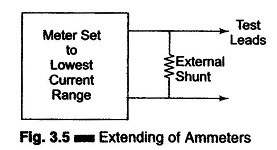Note that the range of the basic meter movement cannot be lowered.

(For example, if a 100 μA movement with 100 scale division is used to measure 1 μA, the meter will deflect by only one division. Hence ranges lower than the basic range are not practically possible.)

Scroll to Top